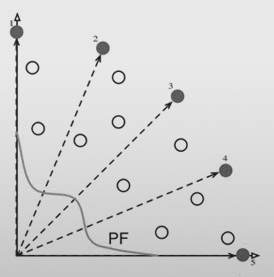# Multi-objective Evolutionary Algorithm by Decomposition (MOEA/D-DE)

Multi Objective Evolutionary Algorithms by Decomposition (the DE variant)MOEA/D-DE is a very successful multi-objective optimization algorithm, always worth a try. Based on the idea of problem decomposition, it leverages evolutionary operators to combine good solutions of neighbouring problems thus allowing for nice convergence properties. MOEA/D is, essentially, a framework and this particular algorithm implemented in pagmo with the name pagmo::moead uses the rand/2/exp Differential Evolution operator followed by a polynomial mutation to create offsprings, and the Tchebycheff, weighted or boundary intersection decomposition method. A diversity preservation mechanism, as proposed in the work from Li et al. referenced below, is also implemented.

Note

The decomposition weights may be created by sampling on a simplex via a low discrepancy sequence. This allows to have MOEA/D-DE work on populations having arbitrary size, while preserving a nice coverage of the final non-dominated front.

Zhang, Qingfu, and Hui Li. “MOEA/D: A multiobjective evolutionary algorithm based on decomposition.” Evolutionary Computation, IEEE Transactions on 11.6 (2007): 712-731.

Li, Hui, and Qingfu Zhang. “Multiobjective optimization problems with complicated Pareto sets, MOEA/D and NSGA-II.” Evolutionary Computation, IEEE Transactions on 13.2 (2009): 284-302.

Public Types

typedef std::tuple<unsigned, unsigned long long, double, vector_double> log_line_type

Single entry of the log (gen, fevals, adf, ideal_point)

typedef std::vector<log_line_type> log_type

The log.

Public Functions

moead(unsigned gen = 1u, std::string weight_generation = "grid", std::string decomposition = "tchebycheff", population::size_type neighbours = 20u, double CR = 1.0, double F = 0.5, double eta_m = 20., double realb = 0.9, unsigned limit = 2u, bool preserve_diversity = true, unsigned seed = pagmo::random_device::next())

Constructor.

Constructs MOEA/D-DE

Parameters
• gen – number of generations

• weight_generation – method used to generate the weights, one of “grid”, “low discrepancy” or “random”

• decomposition – decomposition method: one of “weighted”, “tchebycheff” or “bi”

• neighbours – size of the weight’s neighborhood

• CR – crossover parameter in the Differential Evolution operator

• F – parameter for the Differential Evolution operator

• eta_m – distribution index used by the polynomial mutation

• realb – chance that the neighbourhood is considered at each generation, rather than the whole population (only if preserve_diversity is true)

• limit – maximum number of copies reinserted in the population (only if m_preserve_diversity is true)

• preserve_diversity – when true activates the two diversity preservation mechanisms described in Li, Hui, and Qingfu Zhang paper

• seed – seed used by the internal random number generator (default is random)

Throws

value_error – if gen is negative, weight_generation is not one of the allowed types, realb,cr or f are not in [1.0] or m_eta is < 0, if neighbours is <2

population evolve(population) const

Algorithm evolve method.

Evolves the population for the requested number of generations.

Parameters

pop – population to be evolved

Returns

evolved population

void set_seed(unsigned)

Sets the seed.

Parameters

seed – the seed controlling the algorithm stochastic behaviour

inline unsigned get_seed() const

Gets the seed.

Returns

the seed controlling the algorithm stochastic behaviour

inline void set_verbosity(unsigned level)

Sets the algorithm verbosity.

Sets the verbosity level of the screen output and of the log returned by get_log(). `level` can be:

• 0: no verbosity

• >0: will print and log one line each `level` generations.

Example (verbosity 1):

```Gen:        Fevals:           ADF:        ideal1:        ideal2:
1              0        24.9576       0.117748        2.77748
2             40        19.2461      0.0238826        2.51403
3             80        12.4375      0.0238826        2.51403
4            120        9.08406     0.00389182        2.51403
5            160        7.10407       0.002065        2.51403
6            200        6.11242     0.00205598        2.51403
7            240        8.79749     0.00205598        2.25538
8            280        7.23155    7.33914e-05        2.25538
9            320        6.83249    7.33914e-05        2.25538
10            360        6.55125    7.33914e-05        2.25538
```
Gen, is the generation number, Fevals the number of function evaluation used. ADF is the Average Decomposed Fitness, that is the average across all decomposed problem of the single objective decomposed fitness along the corresponding direction. The ideal point of the current population follows cropped to its 5th component.

Parameters

level – verbosity level

inline unsigned get_verbosity() const

Gets the verbosity level.

Returns

the verbosity level

inline unsigned get_gen() const

Gets the generations.

Returns

the number of generations to evolve for

inline std::string get_name() const

Algorithm name.

One of the optional methods of any user-defined algorithm (UDA).

Returns

a string containing the algorithm name

std::string get_extra_info() const

Extra info.

One of the optional methods of any user-defined algorithm (UDA).

Returns

a string containing extra info on the algorithm

inline const log_type &get_log() const

Get log.

A log containing relevant quantities monitoring the last call to evolve. Each element of the returned `std::vector` is a moead::log_line_type containing: Gen, Fevals, ADR, ideal_point as described in moead::set_verbosity

Returns

an `std::vector` of moead::log_line_type containing the logged values Gen, Fevals, ADR, ideal_point